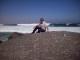# 2 Ways to Calculate Percentage Change in Excel

There are two different ways to calculate percentage change in Excel, and people can be pretty adamant about which way is better. Let me show you both.

## Video Tutorial

First, what do I mean by “percentage change”? I'm just talking about the difference between an old value and a new value expressed as a percent.

For example, if I started with \$5 and I invested it so that now I have \$10, the percentage change would be 100% because I added the entire beginning amount to my balance. On the other hand, if I had lost \$1 of my original \$5, my percentage change would be -20% because 1 is 20% of 5.

## Calculating Percentage Change – Method #1

The first way to go about calculating percentage change is to subtract the old amount from the new amount and then divide that by the old amount.

## Calculating Percentage Change – Method #2

The second way is to divide the new amount by the old amount and then subtract 1.

Both methods will result in the exact same answer, and you'll be able to see the percentage the amount has changed by.

Is one method better than the other? Not really! Method 2 is a little shorter to write because no parentheses are needed. Other than that, there's not much advantage to using one over the other. It's up to you.

•Alda says:

The second method is much faster to write…

•David says:

I default to a/b-1, however (a-b)/b has the advantage of being able to easily modify the formula when calculating the contribution to a total change.

Where the values are component parts of a total and you want to know the impact of a change on the total, you can easily modify to (a-b)/total to get the %pt impact on total change.

•Mike Strong says:

This is a very useful topic and formula. I use methold 1 often.

•Mia Batson says:

I prefer the new/old-1 formula.

•JOSE VASQUEZ TOBAR says:

Los dos metodos son buenos dependeria de en que lo usaria uno o el otro

•Don Hornbrook says:

I’m not comfortable with method #2. It just doesn’t LOOK right, even though it is right. When auditing formulas, much time can be spent tracking down which expressions should be parenthesized and which should not. I’m all for simplifying formulas, but this one could take my attention from the importance of grouping expressions in other formulas. Maybe I’m just lazy.

•Yossi says:

They are algebraically the same.
Prove: (x – y) / y = x/y – 1
(x – y) / y is the same as
1/y * (x – y)
Using the distributive property we get
x/y – y/y
Since y/y = 1 we get
x/y – 1.

•Debbie N-P says:

I am old school…I prefer method 1 [(new-old)/old]. I think it’s because I can understand it….I am not sure of the voodoo magic behind method 2!

•Terri says:

I just use the same formula I learned in grade school – method 1, I never even considered method 2.

•kalpana says:

Wow that was very useful and I think second method is easy but why minus sign

•Bruno says:

What can we do to keep the data clean when the calculation returns ” #DIV/0! “? A temporary solution that I have found so far is ” =IFERROR(((a-b)/a)*100,0) “, which returns zero. I am keen to learn other ways as I’m maintaining a table that calculate these percentage differences however sometimes it can’t be divided as the cell value is zero. Thanks!

•David says:

#1 because the syntax of the formula is clearer, especially for a subsequent user.

•kalpana says:

how do you calculate 4% of \$45321

•Tila BA says:

I prefer the second method.

With the first method, I always forget what should be in the denominator, if it is the old value or the new one.

Generic filters
Exact matches only

#### Excel Shortcuts ListLearn over 270 Excel keyboard & mouse shortcuts for Windows & Mac.

Excel Shortcuts List